Page Card

# Oscillators

Belongs to subject Oscillators

An oscillator creates sound. The sound an oscillator creates is in a simple, repeating cycle. Different types of oscillators produce different kinds of simple sound waves. The five most basic types of oscillators are sine, triangle, square, rectangle, and sawtooth.

# Sine Waves

Sine waves are the simplest sound in the universe. And, sine oscillators are the simplest oscillators. You can create any other oscillator by combining sine oscillators. Sine oscillators take inputs of amplitude and frequency, and sometimes phase. Sometimes sine oscillators are also called circle oscillators.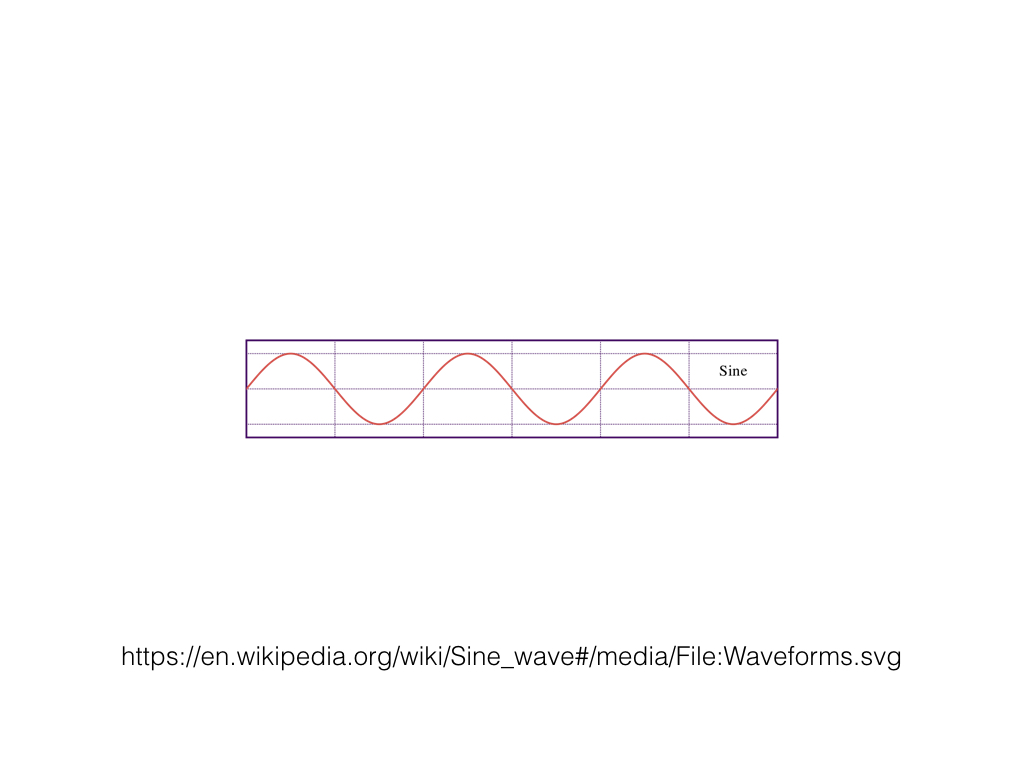# Triangle Waves

Triangle waves are a mathematical combination of sine waves. We make triangle waves from only odd numbered harmonics. This means that above the fundamental frequency, the next is at 3 times the fundamental, then 5 times, and so on. Each of the amplitudes of these is at 1 / (h^2). Triangle waves have a pleasing, gentle sound. We call them "triangle waves" because the shape looks like triangles.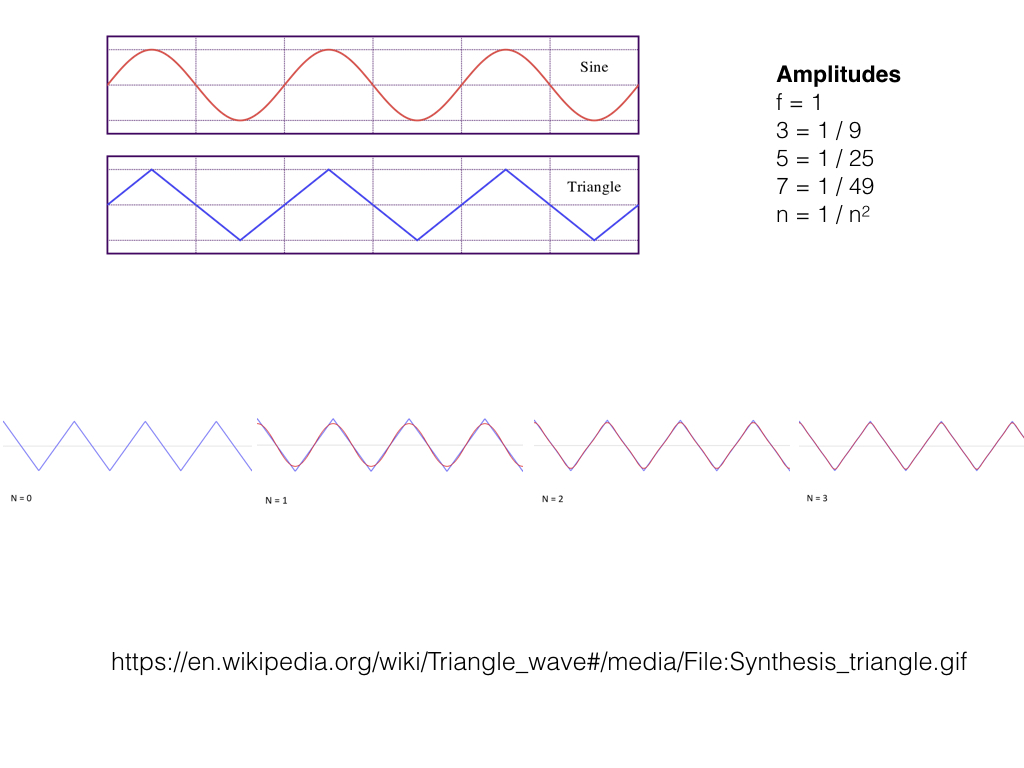# Square Waves

The square wave is like the triangle wave. We make square waves from only odd numbered harmonics just like the triangle wave. Each of the amplitudes of these is at 1 / h instead of 1 / (h^2). This characteristic means square waves have a fuller sound. We call them "square waves" because the shape looks like boxes with 90 degree angles.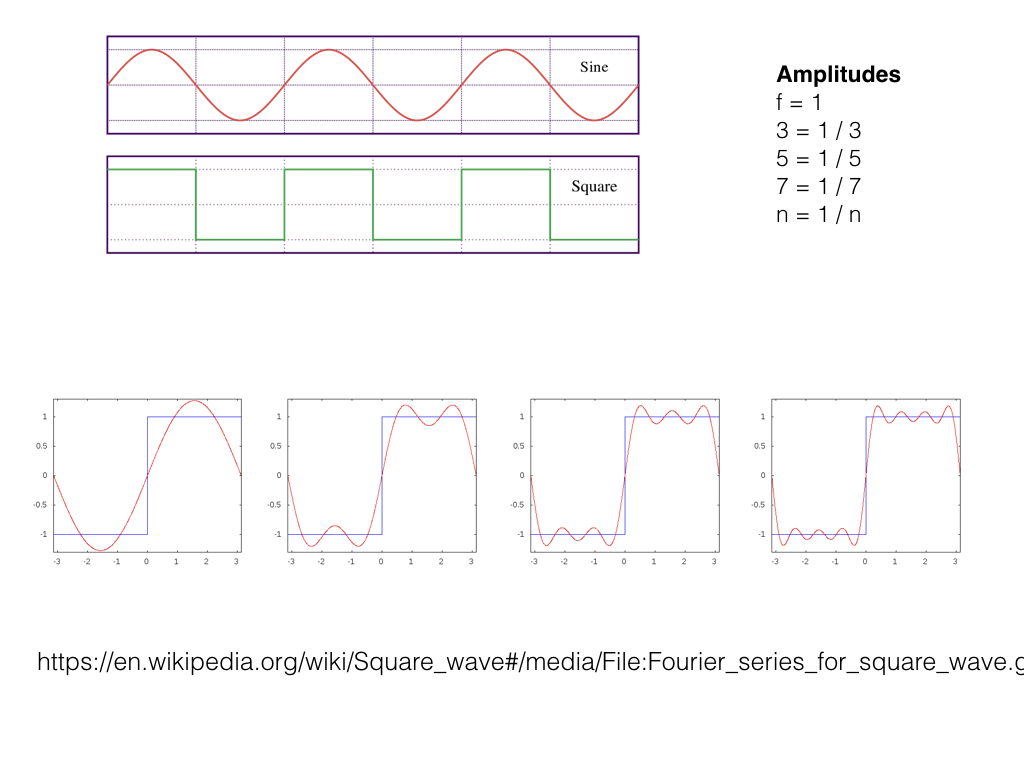# Rectangle Waves

The rectangle wave is the sister of the square wave. The amplitude ratio stays the same at 1 / h. The duty cycle changes which harmonics the oscillator choses to output. The duty cycle informs the oscillator which harmonics to skip. Rectangle waves do not have a pleasing sound. We use rectangle waves often as inputs to other sound producing and changing modules.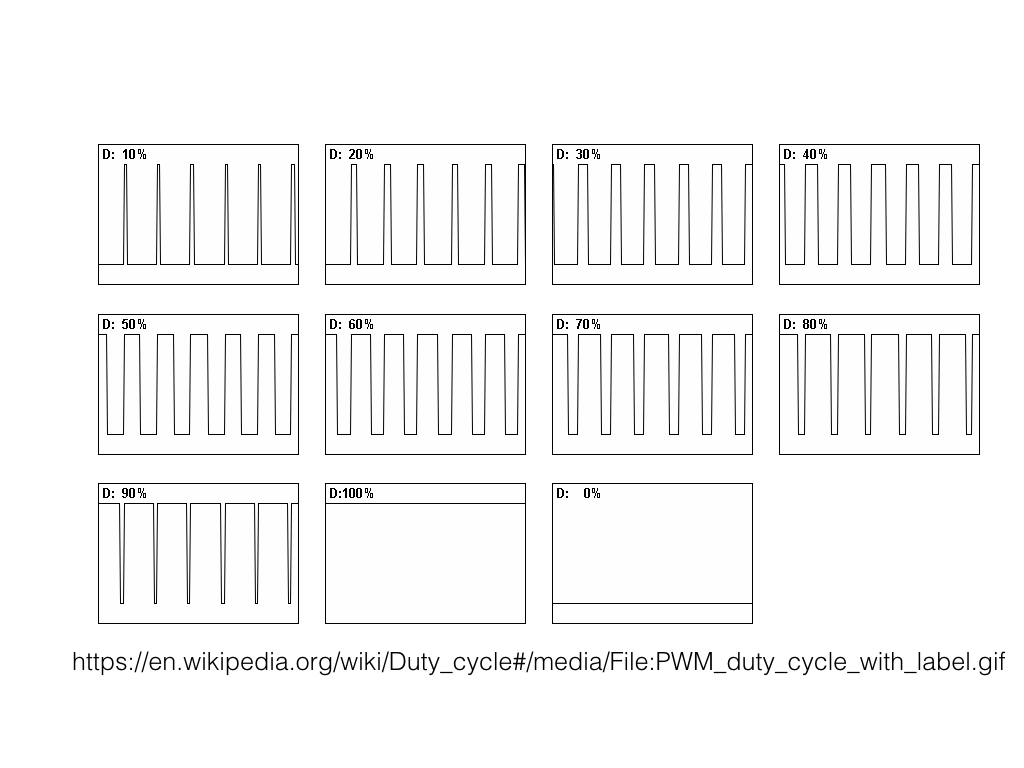# Sawtooth Waves

The sawtooth wave is the richest of the basic oscillators. The sawtooth wave sounds harsh. But, by modifying the sound with filtering, we can produce a wide range of different colors. The amplitude ratio is the same as the square and rectangle wave at 1 / h. But, we include all harmonics, including the even number harmonics. Like the triangle and square wave, we call them "sawtooth waves" because they look like the teeth on a saw.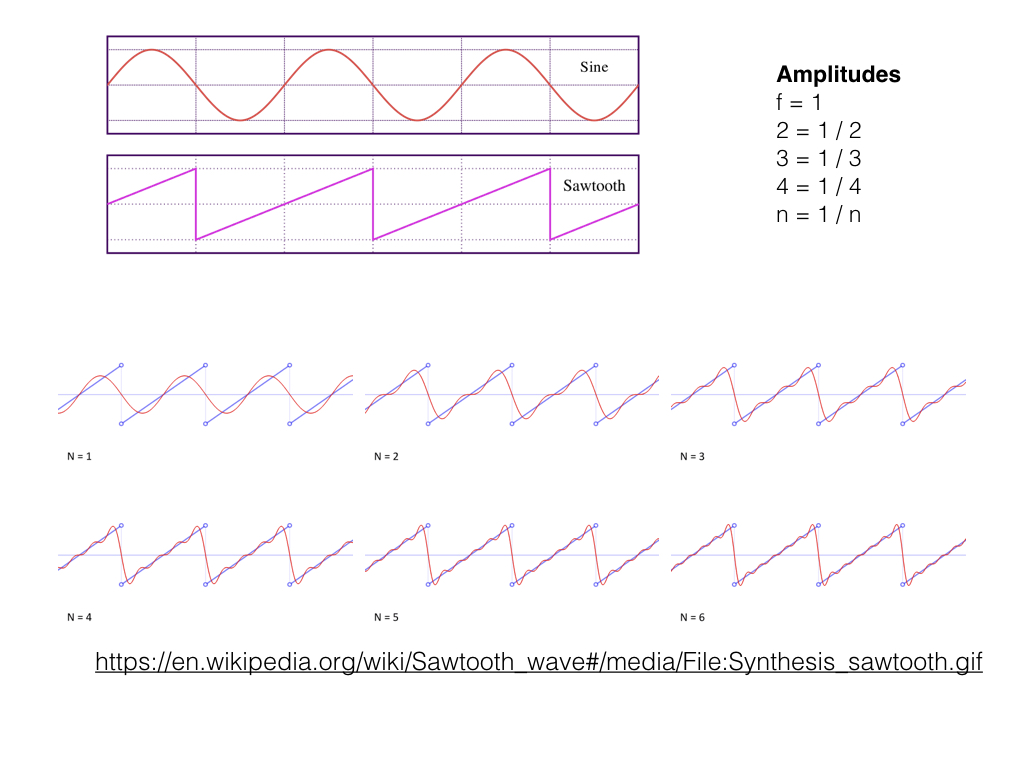These fives types are not the only types of oscillators. There are an infinite number of different types of oscillators, each with different mathematical and sound color properties.